# Volume of Prisms and Cylinders Vocabulary Volume the

• Slides: 16* Volume of Prisms and Cylinders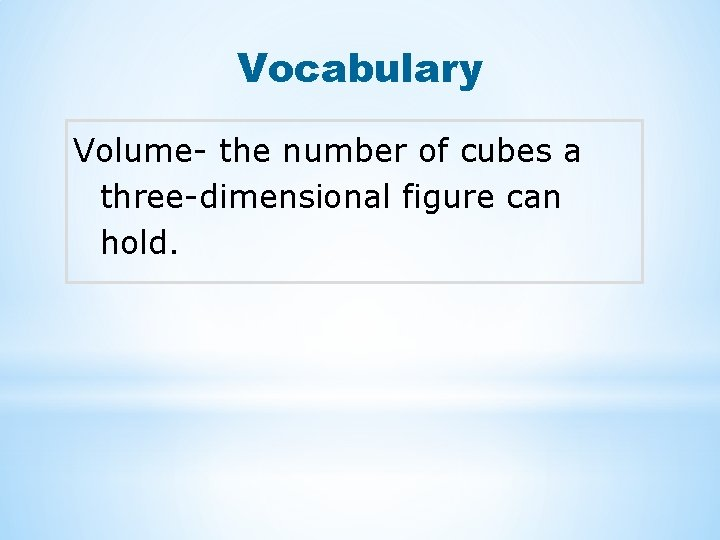Vocabulary Volume- the number of cubes a three-dimensional figure can hold.Any three-dimensional figure can be filled completely with congruent cubes and parts of cubes. The volume of a three-dimensional figure is the number of cubes it can hold. Each cube represents a unit of measure called a cubic unit.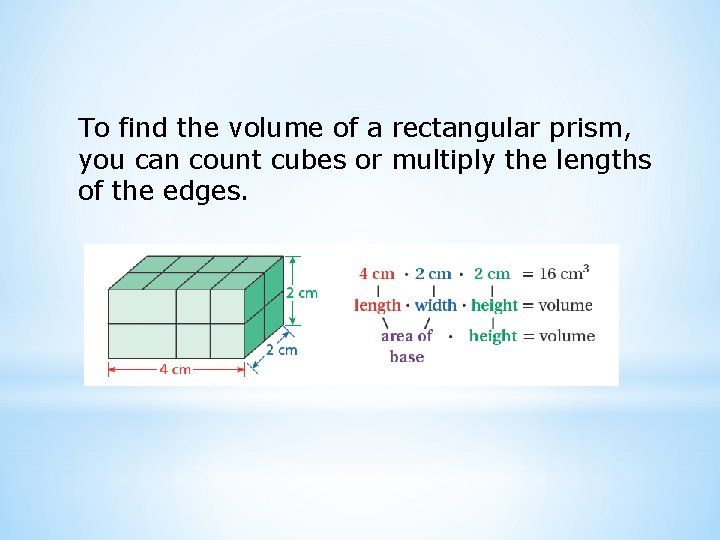To find the volume of a rectangular prism, you can count cubes or multiply the lengths of the edges.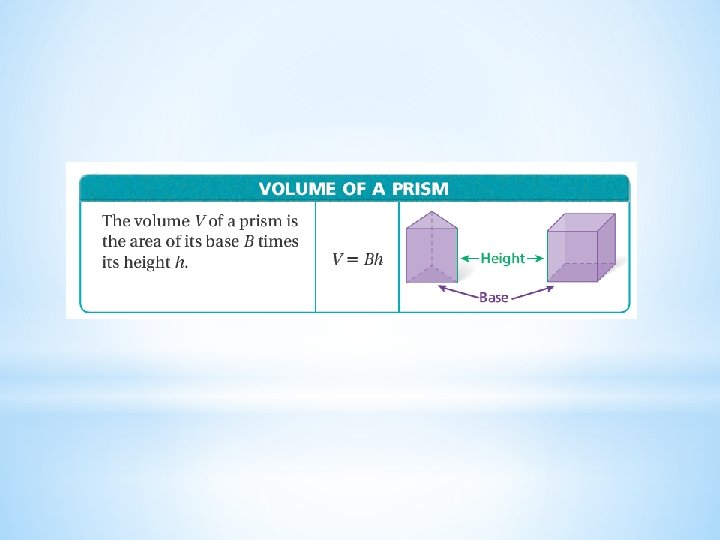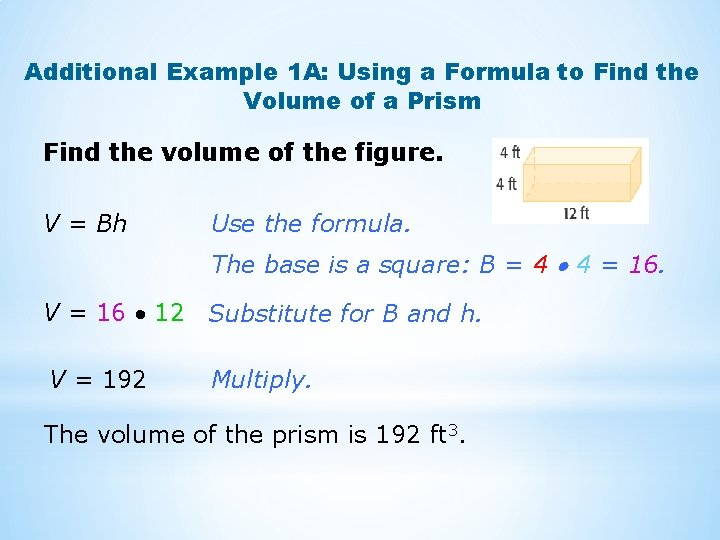Additional Example 1 A: Using a Formula to Find the Volume of a Prism Find the volume of the figure. V = Bh Use the formula. The base is a square: B = 4 4 = 16. V = 16 12 Substitute for B and h. V = 192 Multiply. The volume of the prism is 192 ft 3.Additional Example 1 B: Using a Formula to Find the Volume of a Prism Find the volume of the figure. V = Bh Use the formula. The base is a triangle: B = 1/2 16 6 = 48. V = 48 8 Substitute for B and h. V = 384 Multiply. The volume of the triangular prism is 384 yd 3.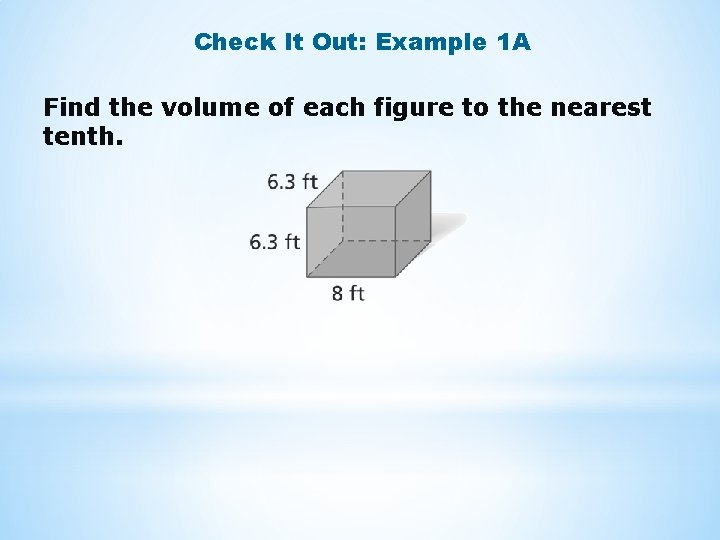Check It Out: Example 1 A Find the volume of each figure to the nearest tenth.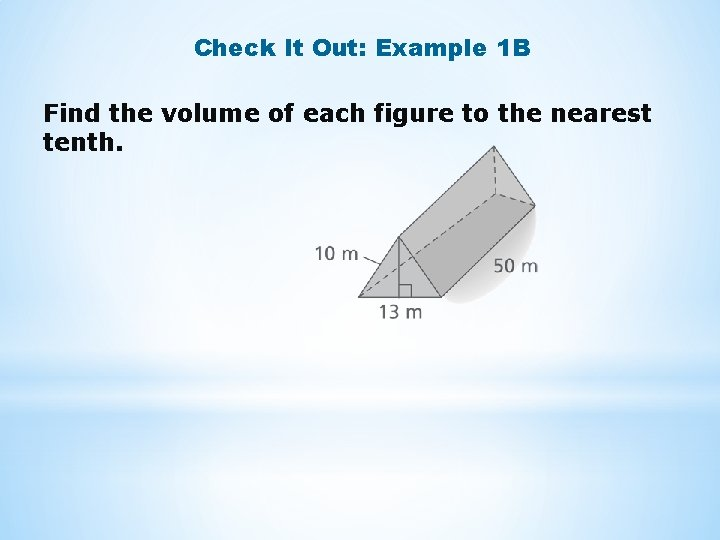Check It Out: Example 1 B Find the volume of each figure to the nearest tenth.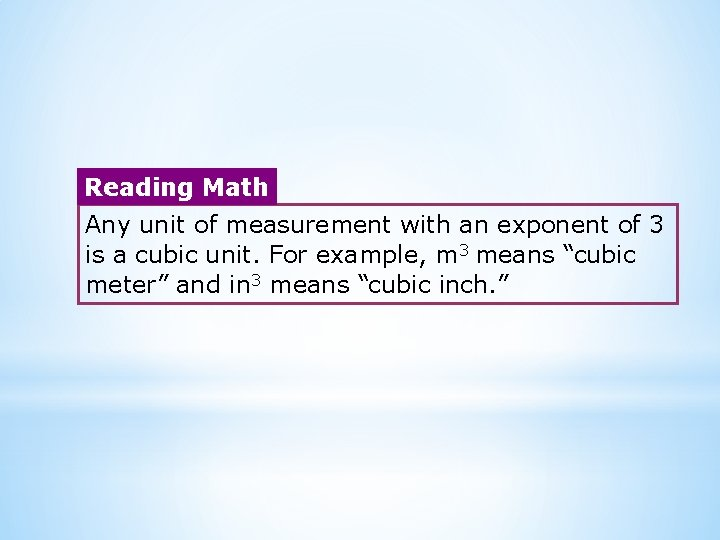Reading Math Any unit of measurement with an exponent of 3 is a cubic unit. For example, m 3 means “cubic meter” and in 3 means “cubic inch. ”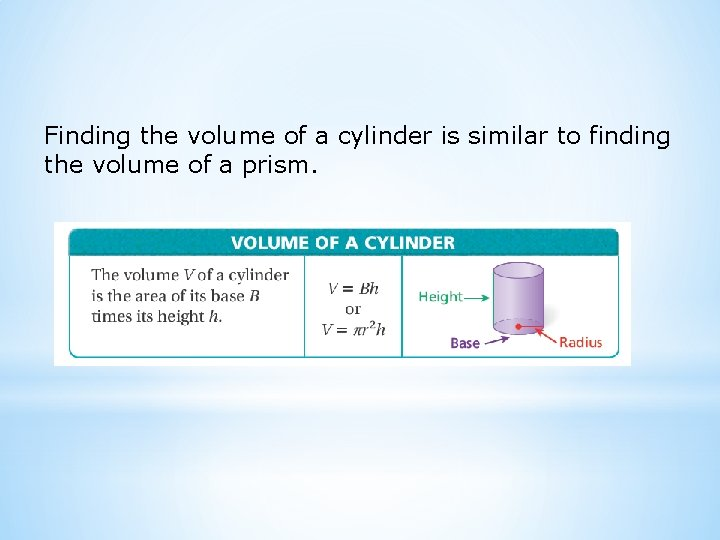Finding the volume of a cylinder is similar to finding the volume of a prism.Additional Example 2: Using a Formula to Find the Volume of a Cylinder A can of tuna is shaped like a cylinder. Find its volume to the nearest tenth. Use 3. 14 for . V = r 2 h Use the formula. The radius of the cylinder is 5 m, and the height is 4. 2 m V 3. 14 · 52 · 4. 2 Substitute for r and h. V 329. 7 Multiply. The volume is about 329. 7 m 3.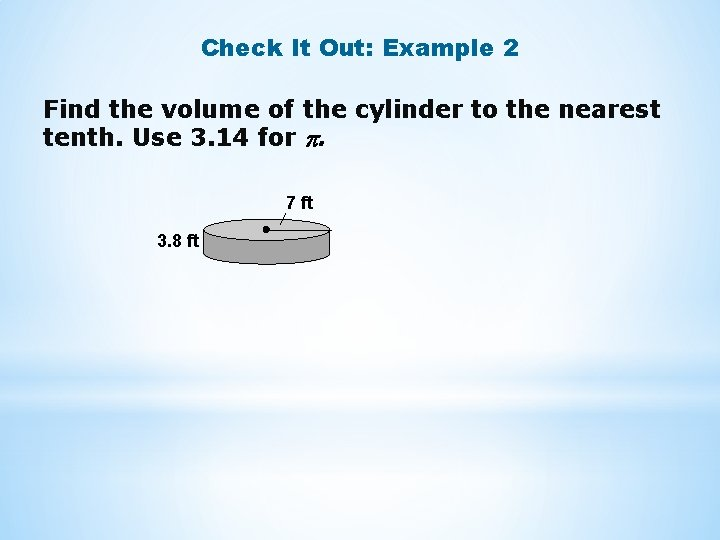Check It Out: Example 2 Find the volume of the cylinder to the nearest tenth. Use 3. 14 for . 7 ft 3. 8 ft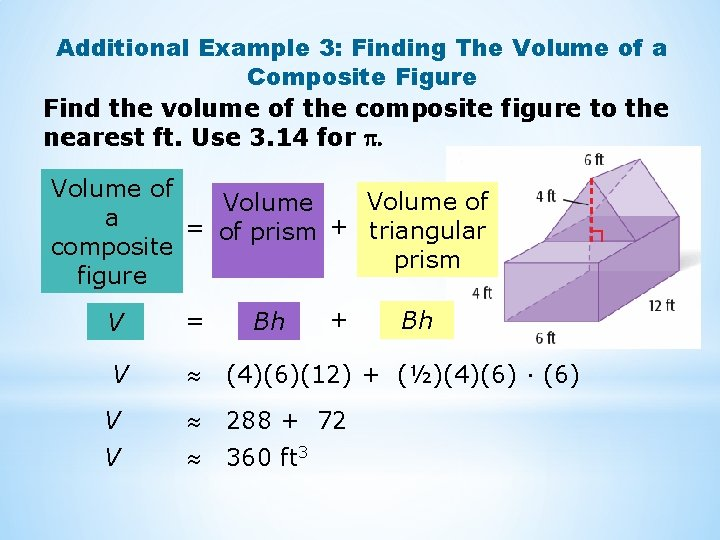Additional Example 3: Finding The Volume of a Composite Figure Find the volume of the composite figure to the nearest ft. Use 3. 14 for . Volume of Volume a = of prism + triangular composite prism figure + V = V (4)(6)(12) + (½)(4)(6) · (6) V 288 + 72 V 360 ft 3 Bh BhCheck It Out: Example 3 A Assume that the prism from Exercise 1 a has been stacked on top of the cylinder from Exercise 2. Find the volume of the composite figure to the nearest tenth. Use 3. 14 for .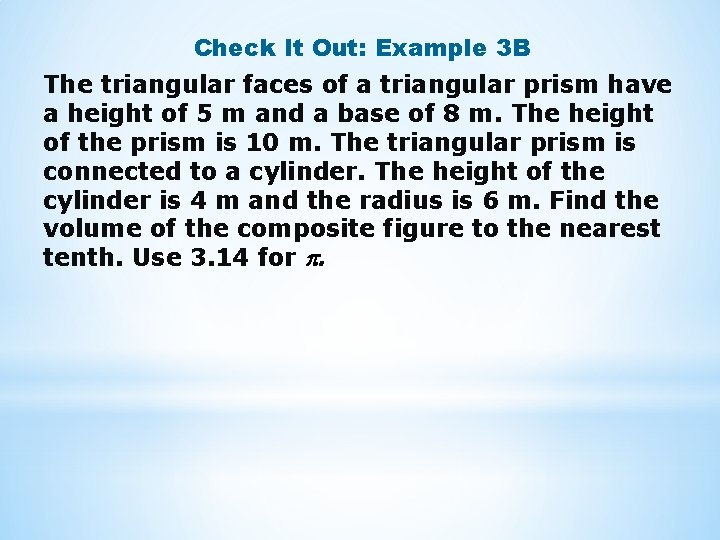Check It Out: Example 3 B The triangular faces of a triangular prism have a height of 5 m and a base of 8 m. The height of the prism is 10 m. The triangular prism is connected to a cylinder. The height of the cylinder is 4 m and the radius is 6 m. Find the volume of the composite figure to the nearest tenth. Use 3. 14 for .